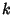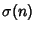## Hyperperfect Number

A numberis called-hyperperfect ifwhere the summation is over the Proper Divisors with, givingwhereis the Divisor Function. The first few hyperperfect numbers are 21, 301, 325, 697, 1333, ... (Sloane's A007592). 2-hyperperfect numbers include 21, 2133, 19521, 176661, ... (Sloane's A007593), and the first 3-hyperperfect number is 325.

References

Guy, R. K. Almost Perfect, Quasi-Perfect, Pseudoperfect, Harmonic, Weird, Multiperfect and Hyperperfect Numbers.'' §B2 in Unsolved Problems in Number Theory, 2nd ed. New York: Springer-Verlag, pp. 45-53, 1994.

Sloane, N. J. A. Sequences A007592/M5113 and A007593/M5121 in An On-Line Version of the Encyclopedia of Integer Sequences.'' http://www.research.att.com/~njas/sequences/eisonline.html and Sloane, N. J. A. and Plouffe, S. The Encyclopedia of Integer Sequences. San Diego: Academic Press, 1995.